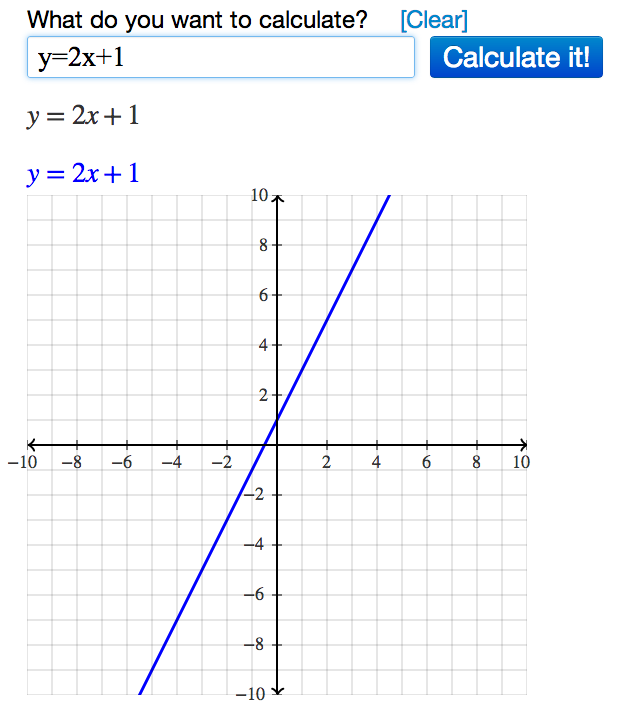# Graphing Equations Using Algebra Calculator

Learn how to use the Algebra Calculator to graph equations.

### Example Problem

Graph the following equation:
y=2x+1

### How to Graph the Equation in Algebra Calculator

First go to the Algebra Calculator main page.

Type the following:

1. y=2x+1
Try it now: y=2x+1

### Clickable Demo

Try entering y=2x+1 into the text box.After you enter the expression, Algebra Calculator will graph the equation y=2x+1.### More Examples

Here are more examples of how to graph equations in Algebra Calculator. Feel free to try them now.